# Running Count of Occurrences in Excel and Google Sheets

In this tutorial, we will learn how to create a running count of values in a range.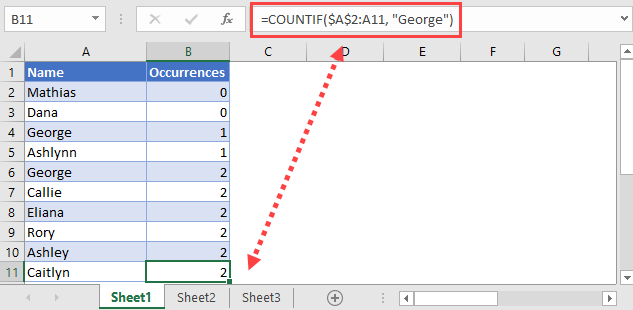## Running Count of Occurrences with COUNTIF and Absolute References

We will be using a combination of the COUNTIF function and absolute references in order to do this.

The COUNTIF function counts all cells in the specified range that meets the condition you have inputted. An absolute reference can be used with the first cell specified to create a running count.

If we specify a range between \$A\$2:A2 and drag that formula down, it will fix the first cell but the second cell will change as we drag it down. As you drag that formula down, the formula’s range will change from \$A\$2:A2 to \$A\$2:A3, \$A\$2:A4 and so on. This is exactly what we need for a running count.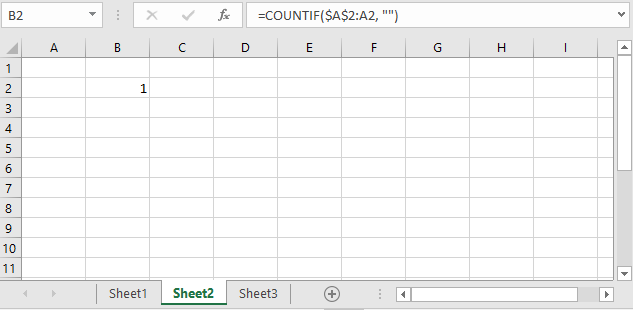TIP: You can highlight a portion of the formula in the formula bar and press F4 to lock the cell/row/column. Pressing F4 once will lock the cell, pressing F4 twice will lock the row, and pressing F4 three times will lock the column (as seen in the GIF below).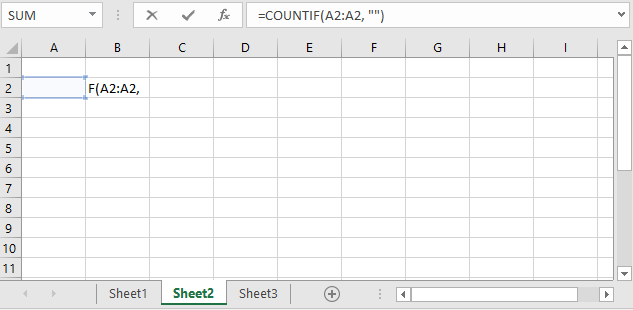Understanding this allows us to create a running count of occurrences for a condition that we specify. For example, see the data set below.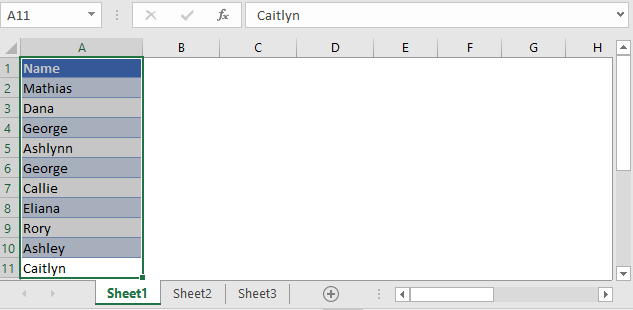Let’s say in this data set, we want to find the number of times the name “George” shows up.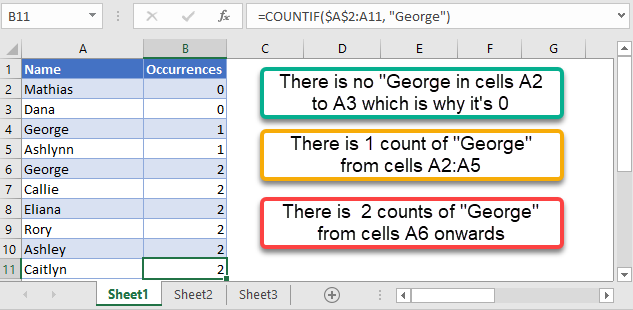``=COUNTIF(Range, Text) [Where the first cell in the range is fixed/absolute]``
``=COUNTIF(\$A\$2:A2, “George”) [Drag the formula down]``

You can see that between cells A2:A3, there is a 0 count of the name “George”.

In cell A4, the name “George” is found in the range which is why the value in B4 now changes to 1.

It changes to 2 from cell B6 onwards because the name “George” is found in the range a second time in cell A6.

## Running Count of Occurrences in Google Sheets

This function works the same way in Google Sheets as well.

``=COUNTIF(Range, Text) [Where the first cell in the range is fixed/absolute]``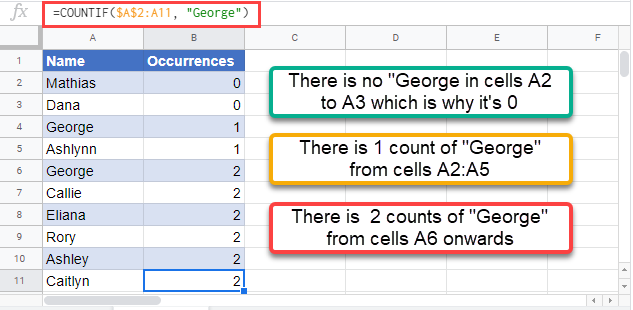### Excel Practice Worksheet

Practice Excel functions and formulas with our 100% free practice worksheets!